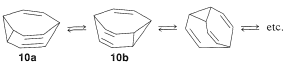# 22.13: Fluxional Compounds

$$\newcommand{\vecs}{\overset { \rightharpoonup} {\mathbf{#1}} }$$ $$\newcommand{\vecd}{\overset{-\!-\!\rightharpoonup}{\vphantom{a}\smash {#1}}}$$$$\newcommand{\id}{\mathrm{id}}$$ $$\newcommand{\Span}{\mathrm{span}}$$ $$\newcommand{\kernel}{\mathrm{null}\,}$$ $$\newcommand{\range}{\mathrm{range}\,}$$ $$\newcommand{\RealPart}{\mathrm{Re}}$$ $$\newcommand{\ImaginaryPart}{\mathrm{Im}}$$ $$\newcommand{\Argument}{\mathrm{Arg}}$$ $$\newcommand{\norm}{\| #1 \|}$$ $$\newcommand{\inner}{\langle #1, #2 \rangle}$$ $$\newcommand{\Span}{\mathrm{span}}$$ $$\newcommand{\id}{\mathrm{id}}$$ $$\newcommand{\Span}{\mathrm{span}}$$ $$\newcommand{\kernel}{\mathrm{null}\,}$$ $$\newcommand{\range}{\mathrm{range}\,}$$ $$\newcommand{\RealPart}{\mathrm{Re}}$$ $$\newcommand{\ImaginaryPart}{\mathrm{Im}}$$ $$\newcommand{\Argument}{\mathrm{Arg}}$$ $$\newcommand{\norm}{\| #1 \|}$$ $$\newcommand{\inner}{\langle #1, #2 \rangle}$$ $$\newcommand{\Span}{\mathrm{span}}$$$$\newcommand{\AA}{\unicode[.8,0]{x212B}}$$

A number of compounds are known to rearrange from one structure to an entirely equivalent structure, sometimes with extraordinary facility. Such compounds are said to be fluxional to distinguish from tautomers (which usually involve rearrangements between nonequivalent structures). Simple examples are the Cope rearrangement of 1,5-hexadiene,and the electrocyclic rearrangement of bicyclo[5.1.0]-2,5-octadiene,If the two methylenes of $$9$$ are bridged with two double-bonded carbons, we get the remarkable structure $$10$$, called "bullvalene", which rapidly interconverts amongst equivalent structures:Equilibration of fluxional molecules must not be confused with resonance. In each electrocyclic reaction, the nuclei alter their positions as bond lengths and angles change. Interconversion of fluxional molecules also must not be confused with conformational changes (as in the interconversion of two equivalent chair forms of cyclohexane). In the interconversion of fluxional molecules, chemical bonds are broken and made; in conformational changes, no bonds are broken and no bonds are made.

Equilibration of fluxional molecules must not be confused with resonance.

A theoretical prediction is that the very-large-ring annulenes, even those with $$\left[ 4n + 2 \right]$$ $$\pi$$ electrons, may not have sufficient resonance energy to maintain equal bond lengths between the carbons and hence would be most stable with alternating double and single bonds. These substances then would be as Kekule thought benzene to be - a fluxional, equilibrating mixture of cyclohexatrienes! This does not mean that there would be no $$\pi$$-electron delocalization in fluxional cyclic polyenes; it means only that the VB structures would not be exactly equivalent and the MO model would have a $$\sigma$$-bond framework with alternating short and long $$\ce{C-C}$$ bonds. The theory suggests that if alternation in bond lengths occurs, then neither diamagnetic nor paramagnetic circulation of the $$\pi$$ electrons should be important. The synthesis and study of - and annulenes seems to bear out this prediction, in that with these substances there appears to be no ring-current effect on the proton chemical shifts.